Next:3.6.2 Result 2: InsertionUp:3.6 Analysis of Closed HashingPrevious:3.6 Analysis of Closed Hashing

## 3.6.1 Result 1: Unsuccessful Search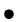The expected number of probes in an unsuccessful search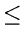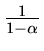Proof: In an unsuccessful search, every probe but the last accesses an occupied slot that does not contain the desired key and the last slot probed is empty.

Let the random variable X denote the number of occupied slots probed before hitting an empty slot. X can take values 0, 1,..., n. It is easy to see that the expected number of probes in an unsuccessful search is 1 + E[X].

 pi = P{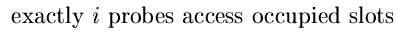}, for i = 0, 1, 2,...

for i > n, pi = 0 since we can find at most n occupied slots.

Thus the expected number of probes
= 1 +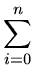ipi                                                 (*)
To evaluate (*), define

qi = P{at least  i  probes access occupied slots}
and use the identity:ipi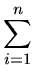qi
To compute qi, we proceed as follows:
q1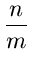since the probability that the first probe accesses an occupied slot is n/m.

With uniform hashing, a second probe, if necessary, is to one of the remaining m - 1 unprobed slots, n - 1 of which are occupied. Thus
q2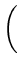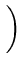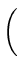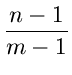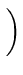since we make a second probe only if the first probe accesses an occupied slot.

In general,

 qi =...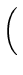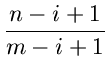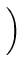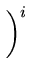=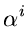since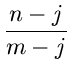Thus the expected number of probes in an unsuccessful search

 = 1 +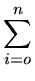ipi1 +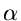+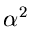+ ... =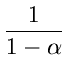Intuitive Interpretation of the above:

One probe is always made, with probability approximatelya second probe is needed, with probability approximately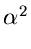a third probe is needed, and so on.Next:3.6.2 Result 2: InsertionUp:3.6 Analysis of Closed HashingPrevious:3.6 Analysis of Closed Hashing
eEL,CSA_Dept,IISc,Bangalore